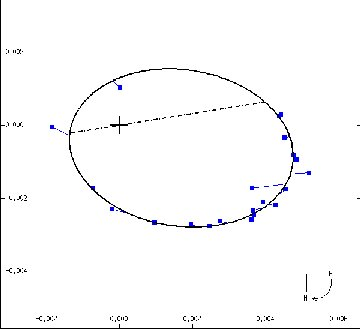THETA AQL (Theta Aquilae). Project a line from Altair (the luminary of Aquila, the Eagle) through its southern outlier Alshain (Beta Aquilae), and it passes almost straight through third magnitude (3.23) Theta Aquilae. To the east of the main line of the Milky Way, the star marks a sharp westward bend in the constellation's classic outline. As a distance of 287 light years, This seemingly ordinary class B (B9.5, just to the high temperature side of Vega-like class A0) giant hides a surprise. It's not just one B9.5 star, but two of them (as best we can tell the classes) very close together. Moreover, as seen below, neither of them is a giant. The spectrograph reveals the pair in tight orbital motion with an orbital period of a mere 17.123 days. Too close together to separate visually at the telescope (an astoundingly small 0.004 seconds of arc), the stars have been individually "seen" through the extraordinary power of "interferometry," which makes use of the interfering properties of light waves.The interferometer reveals the tight orbit of fifth magnitude Theta Aquilae B as it orbits third magnitude Theta Aql A, whjich lies at the cross (in reality the two orbiting a common center of mass between them). The numbered marks on the axes (hard to read here) are just 0.002 seconds of arc apart. A fit of an ellipse to the partially observations (coupled with spectroscopy) gives an orbital period of 17.123 days and an average orbital size of 0.28 AU (which from theoretical analysis of the stars themselves is probably more like 0.24 AU). The elliptical orbit is seen as tilted by 36 degrees to the plane of the sky. (From an article by C. A. Hummel et al. in the Astronomical Journal, vol. 110, p. 376, 1995, as given in the Sixth Catalog of Orbits of Visual Binary Stars, W. I. Hartkopf and B. D. Mason, U.S. Naval Observatory.)
Theta A comes in at the lower end of third magnitude (3.47), while Theta B is nailed right at exact fifth (5.00). Assuming that the temperature of 10,800 Kelvin measured for Theta A also applies to B (needed to allow for a bit of ultraviolet light), they have respective luminosities of 370 and 90 times that of the Sun, which yield radii 5.5 and 2.7 solar. Application of theory tells of masses of 3.7 and 2.8 solar and that Theta A is more properly a subgiant right at, or near, the end of its hydrogen-fusing lifetime, whereas Theta B is a middle-aged dwarf, the stars about 200 million years old. Interferometric observation of the orbiting pair gives an average orbital separation of 0.28 Astronomical Units, just three quarters of Mercury's distance from the Sun. Kepler's laws then give a sum of masses of 10.2 times solar, considerably more than the 6.5 given by evolutionary theory. A small adjustment down to a 0.24 AU orbital radius reconciles the two, however. A relatively high eccentricity (rather odd for stars this close together) takes them between 0.10 and 0.39 AU apart. The projected equatorial rotation speed of 64 kilometers per second for Theta A leads to a rotation period under 4.9 days. Assuming the axial tilt is the same as that of the orbit, the true rotation period is but 2.9 days. Unlike many class B stars, this one (Theta A) is metal-rich, with an iron-to-hydrogen ratio 60 percent greater than solar. Nearly two minutes of arc away is 13th magnitude Theta Aql C, which -- if a real relative -- would be a class K dwarf 10,000 AU from the main pair and have an orbital period greater than 300,000 years. Most likely, though, it is just another line- of-sight coincidence. More interesting, the star seems to be embedded in a large reflection nebula (its dust reflecting the starlight) that -- if the two are indeed related -- is some five light years across.